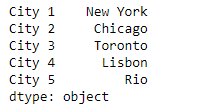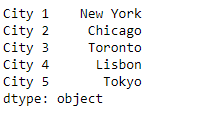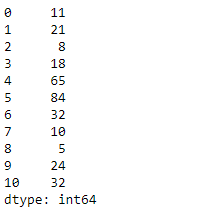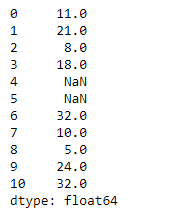• Last Updated : 11 Feb, 2019

Pandas series is a One-dimensional ndarray with axis labels. The labels need not be unique but must be a hashable type. The object supports both integer- and label-based indexing and provides a host of methods for performing operations involving the index.

Pandas` Series.mask()` function is used for masking purpose. This function replace values where the passed condition is `True`. Otherwise the value remains same.

Syntax: Series.mask(cond, other=nan, inplace=False, axis=None, level=None, errors=’raise’, try_cast=False, raise_on_error=None)

Parameter :
cond : Where cond is False, keep the original value. Where True, replace with corresponding value from other.
other : Entries where cond is True are replaced with corresponding value from other.
inplace : Whether to perform the operation in place on the data.
axis : Alignment axis if needed.
level : Alignment level if needed.

Returns : wh : same type as caller

Example #1: Use `Series.mask()` function to replace the ‘Rio’ city in the given series object.

 `# importing pandas as pd``import` `pandas as pd`` ` `# Creating the Series``sr ``=` `pd.Series([``'New York'``, ``'Chicago'``, ``'Toronto'``, ``'Lisbon'``, ``'Rio'``])`` ` `# Create the Index``index_ ``=` `[``'City 1'``, ``'City 2'``, ``'City 3'``, ``'City 4'``, ``'City 5'``] `` ` `# set the index``sr.index ``=` `index_`` ` `# Print the series``print``(sr)`

Output :Now we will use `Series.mask()` function to replace the ‘Rio’ city in the given series object.

 `# replace 'Rio' with 'Tokyo'``result ``=` `sr.mask(``lambda` `x : x ``=``=``'Rio'``, other ``=` `'Tokyo'``)`` ` `# Print the result``print``(result)`

Output :As we can see in the output, the `Series.mask()` function has successfully replaced the ‘Rio’ city with ‘Tokyo’ in the given series object.

Example #2: Use `Series.mask()` function to mask all the values in the given series object which are greater than 50.

 `# importing pandas as pd``import` `pandas as pd`` ` `# Creating the Series``sr ``=` `pd.Series([``11``, ``21``, ``8``, ``18``, ``65``, ``84``, ``32``, ``10``, ``5``, ``24``, ``32``])`` ` `# Print the series``print``(sr)`

Output :Now we will use `Series.mask()` function to mask all the values greater than 50 in the given series object.

 `# mask values greater than 50``result ``=` `sr.mask(sr > ``50``)`` ` `# Print the result``print``(result)`

Output :As we can see in the output, the `Series.mask()` function has successfully masked all the values greater than 50 in the given series object.

My Personal Notes arrow_drop_up